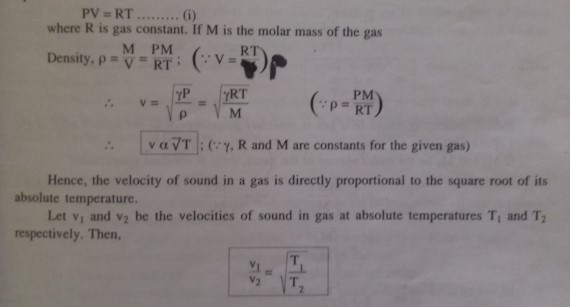Factor affecting the Velocity of Sound in a GasFactors Affecting the Velocity of Sound in a Gas

(a) Effect of temperature: For one mole of an ideal gas having pressure P and volume V, the equation of state is,(b) Effect of pressure: For one mole of gas, equations of state isThus, if temperature of a gas remains constant, the change in pressure of the gas changes its density in the same ratio, so (P/ρ) remains unchanged. Hence, the velocity of sound in a gas is independent of the pressure of the gas provided the temperature remains constant.

(e) Effect of density: v1 and v2 be the velocities of sound in gases of densities ρ1, and ρrespectively. If γ and P are same for both, thenHence, velocity of sound in a gas is inversely proportional to the square root of the density of the gas.

If M1, and M2 be the molar masses of the gases, then, at constant temperature,Thus, velocity of sound is inversely proportional to the square root of molecular weight of the gas.

(d) Effect of humidity: The density of water vapour is less than the density of dry air. Therefore, density of humid air (containing more water vapour) is less than the density of dry air. Since, the velocity of sound is inversely proportional to the square root of density of the gas, sound travels faster in humid (or moist) air than in dry air. Due to the same reason, sound is heard up to longer distances during rainy days.

(e) Effect of wind: As sound travels in the air medium, the motion of the medium affects the velocity of sound. If the medium (air) carrying the sound wave is moving, then the speed of sound is given by the resultant of their speeds. Thus, if both sound and air are moving in the same direction, then resultant velocity of sound is equal to the sum of velocities of sound and air. Similarly, if wind is blowing in the direction opposite to the direction of sound, the resultant velocity of sound is equal to the difference of their velocities.